## Example Questions

### Example Question #1 : Lowest Common Denominator

What is the difference between the LCM and GCF for the following set of numbers:  3, 12, and 30?

None of the answers are correct

57

75

48

60

57

Explanation:

LCM = least common multiple = 2 x 2 x 3 x 5 = 60

GCF = greatest common factor = 3

Prime factor each number

3 = 3 x 1

12 = 3 x 4 = 3 x 2 x 2

30 = 5 x 6 = 5 x 2 x 3

LCM – GCF = 60 – 3 = 57

### Example Question #2 : Lowest Common Denominator

What is the least common denominator of,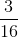, and?

24

48

16

32

16

Explanation:

In order to find the least common denominator, you must find the least common multiple of all three numbers.  For this problem, the least common multiple of all three numbers is 16 (divisible by 2, 4, 8 , 1, and 16).

### Example Question #3 : Lowest Common Denominator

What is the lowest common denominator for the following five fractions?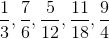12

36

3

18

6

36

Explanation:

The lowest common denominator looks at the denominator (bottom number) of all of the fractions and finds the smallest number that all of the numbers divide into.

Of those numbers the largest is 18.  First check to see if all the numbers divide into 18.  3 and 6 do, but 4 and 12 do not.  Multiply 18 by 2 and get 36.  Check to see if all the numbers divide into 36.  3 and 6 still do.  4 and 12 do now. therefore. 36 is the lowest common denominator.

### Example Question #4 : Lowest Common Denominator

Find the lowest common denominator of these four fractions: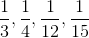45

60

15

30

24

60

Explanation:

All of the denominators must be able to divide into the same number. First, see if the three smaller numbers (3, 4, 12) divide into the largest number (15)—NO. Then check the multiples of the largest number to see if the lower numbers divide into it:

15 * 2 = 30 (NO)

15 * 3 = 45 (NO)

15 * 4 = 60 (YES!)

### Example Question #5 : Lowest Common Denominator

Solve the following: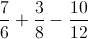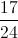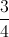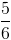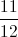Explanation:

Finding the common denomenator ofyields a result of### Example Question #6 : Lowest Common Denominator

Find the least common denominator for the following fractions: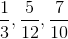120

360

30

60

36